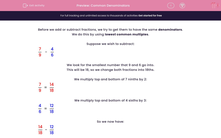# Common Denominators

In this worksheet, students use common multiples to express fractions in the same denominators.Key stage:  KS 2

Curriculum topic:   Maths and Numerical Reasoning

Curriculum subtopic:   Fractions

Difficulty level:#### Worksheet Overview

Before we add or subtract fractions, we try to get them to have the same denominators.

We do this by using lowest common multiples.

Suppose we wish to subtract:

 7 - 4 9 6

We look for the smallest number that 9 and 6 go into.

This will be 18, so we change both fractions into 18ths.

We multiply top and bottom of 7 ninths by 2:

 7 = 14 9 18

We multiply top and bottom of 4 sixths by 3:

 4 = 12 6 18

So we now have:

 14 - 12 18 18

 2 = 1 18 9

Example

When we work out this calculation, what is the lowest common denominator we should choose for both fractions?

 7 - 4 15 10